Next: References Up: Breit-Wheeler Process Previous: Usage

### Algorithm

Because of the threshold behavior, the algorithm is slightly different from that for the Compton process. The mesh for q cannot be equally spaced. Select q's such that all the threshold points 1/n (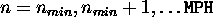,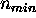= integer part of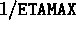) are included, that q are equally spaced between successive thresholds, and that the spaces are not very diffrent. Thus, the total number of q's may not exactly equal to MQ.

The functions stored in the array GG(k,n,i,j,l) are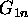(k=1) and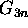(k=2). The integral GINT(k,n,i,j,l) is calculated as in the Compton case. The sum of GINT(k,n,MY,j,l) over n does not make sense because the result would be a quite discontinuos function of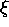and q, which makes the interpolation inaccurate. However, for given q, the sum from n=n(q) to MPH is continuous where n(q) is the minimum integer which does not exceed 1/q. Thus, the sum over n=n to MPH is stored in GALL(k,n,j,l).

For given initial condition, calculateand q. Then, interpolate GALL(k,n(q),j,l) for j and l and calculate the total probability P by summing for appropriate polarization: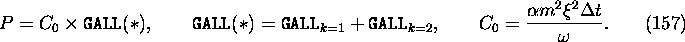Generate a uniform random number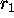and reject an event if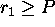. In this case the helicity of the photon should change to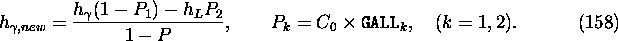If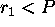, decide to create a pair. The number of laser photons to absorb is determined from GINT(*,n,MY,j,l) as in the Compton case.

To determine the electron energy, generate another random number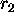in the range (-1,+1). Then, y (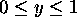) is determined from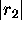as in the Compton case. Adopt -y instead of y if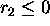.

The helicity of electron is given by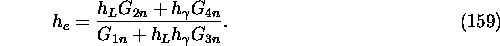The positron momentum is calculated by the momentum conservation and the helicity from the above formula with y replaced by -y.

Toshiaki Tauchi
Thu Dec 3 17:27:26 JST 1998